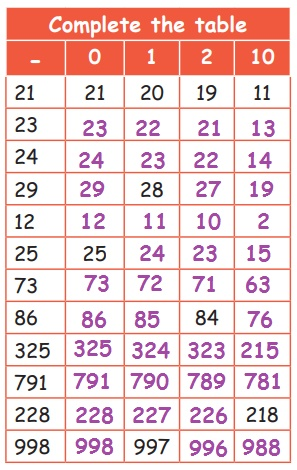Home | | Maths 3rd Std | Quick Ways of subtracting

# Quick Ways of subtracting

We shall learn some tricks in subtraction also.

Quick Ways of subtracting

We shall learn some tricks in subtraction also.

1. We shall use the subtraction table to subtract small numbers2. Some facts in subtraction

subtracting 0 from a number

A number remains the same when you subtract 0 from it

Subtracting 1 from a number

When 1 is subtracted from a number, it gives a number before that number.

Subtracting 2 from a number

When 2 is Subtracted from a number, the numbers moves backward 2 steps

Subtracting 10 from a number

When 10 is Subtracted from a number its ones place remains the same and digit in 10 place is decreased by 1 i.e., moves to the previous number.

3. Subtracting same numbers

Subtracting a number from itself will give the difference 0.

Example

Find the difference of 978−978 = 0

4. Subtracting numbers ending with 0

Subtract 1 from both numbers and then do the actual subtraction

Example

Find the difference of 340 − 229Example

Find the difference of 1000−574As we subtract 1 from both the numbers the answer of the new numbers and question are same.

Subtract the given numbers using the above facts.Tags : Information Processing | Term 3 Chapter 7 | 3rd Maths , 3rd Maths : Term 3 Unit 7 : Information Processing
Study Material, Lecturing Notes, Assignment, Reference, Wiki description explanation, brief detail
3rd Maths : Term 3 Unit 7 : Information Processing : Quick Ways of subtracting | Information Processing | Term 3 Chapter 7 | 3rd Maths